# How to Count Cells Less Than a Specific Value in Excel

This post will guide you how to count the number of cells less than a particular numeric value in a given range cells using a formula in Excel 2013/2016. How do I count cells that are less than a specific value through VBA Macro in Excel. How to count cells if value is less than a particular value using COUNTIF function in Excel.

## Count Cells Less Than a Specific Value

Assuming that you are working with long data sheet, and you need to count the number of cells that have values if only the value in the cell is less than a particular number (`70`). Or you want to count values where data value of the corresponding values is less than a given cell value. In this case, you can use the COUNTIF function to count the number of cells in range `B2:B6` that have a value less than the value (70) or in cell D1. Using the following formula:

=COUNTIF(B2:B6,”<“&D1)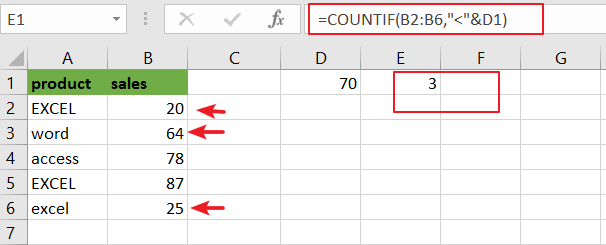Or you can also use the COUNTIF function to count the number of cells that contain a value of less than a specific value (70), which is directly entered into the formula, type:

=COUNTIF(B2:B6,”<70″)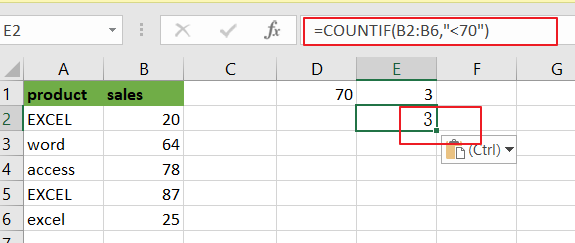Note: for the above formula, `B2:B6` is a range of cells that you want to use, and “`<70`” is the criteria above which you want to count. You also need to know that the COUNTIF function is not case-sensitive.

Now Let’s see the syntax of the COUNTIF Function:

The COUNTIF function is a built-in function. It can be used to do several calculations to find out exactly how many cells in a given range that have a value of less than the value that you have specified.

=COUNTIF (Range, Criteria)

This function has two arguments, range and criteria, which are required to get the exact result.

Range: when one or more than one cell is taken into account for counting operation, then this is regarded as a range.

Criteria: it is a specific condition that you want to specify to let the function know which cells you want to take into account for counting. It could be any text string, number, cell reference or an array list.

Note: you need to know that the COUNTIF function is not case sensitive. And if you want to count the number of cells in a range with case-sensitive, you need to use count cells lessr than 3.

## Count Cells Less Than a Specific Value Through VBA

To count the number of cells in the range that contain numeric values less than a specific value, and you can use the Excel VBA Macro to achieve the same result. Just do the following steps:

Step1# open your excel workbook and then click on “Visual Basic” command under DEVELOPER Tab, or just press “ALT+F11” shortcut.Step2#  then the “Visual Basic Editor” window will appear.

Step3# click “Insert” ->”Module” to create a new module.Step4# paste the below VBA code into the code window. Then clicking “Save” button.

```Sub CountCellLessThanTo()

Dim mysheet As Worksheet

Set mysheet = Worksheets("Sheet7")

mysheet.Range("E1") = Application.WorksheetFunction.CountIf(mysheet.Range("B2:B6"), "<=" & mysheet.Range("D1"))

End Sub```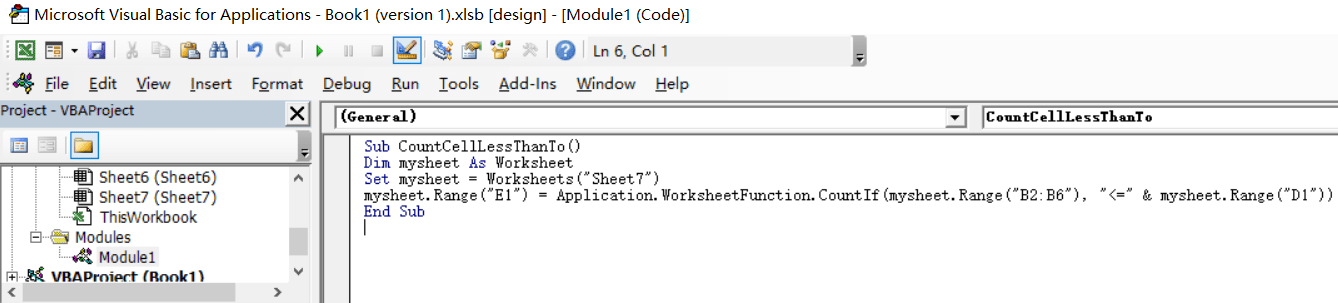Step5: back to the current worksheet, click on `Macros` button under `Code` group. then click `Run` button.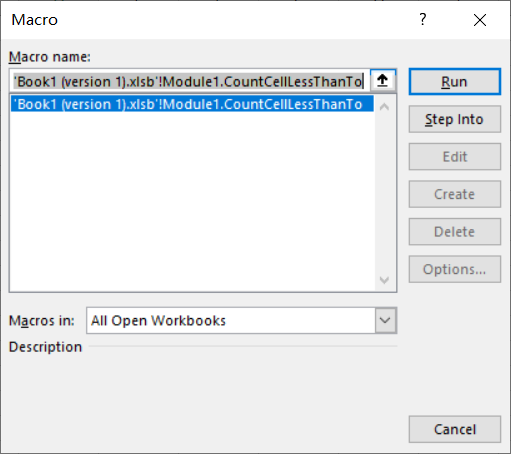Step6: let’s see the result: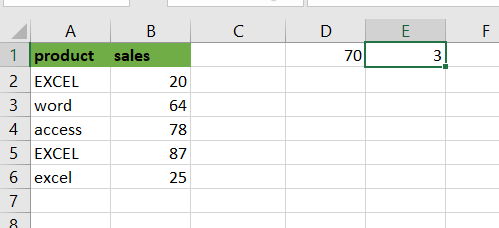Note:

Cell `E1` is the output range, you need to change the cell reference E1 as you need in the VBA code.

`Sheet7` is the worksheet name, and you need to change the name of “mysheet” object variable as you need.

`B2:B6` is the range from which you want to count cells that are less than a particular cell value in cell D1.

`D1` is a specific value that you want to count if a cell’s value is less than to the value in Cell D1.

If you want to count the cells that are less than a specific value with the value entered directly into the VBA code, you can use the following VBA code:

```Sub CountCellLessThanTo()

Dim mysheet As Worksheet

Set mysheet = Worksheets("Sheet7")

mysheet.Range("E1") = Application.WorksheetFunction.CountIf(mysheet.Range("B2:B6"), "<=70")

End Sub```

### Related Functions

• Excel COUNTIF function
The Excel COUNTIF function will count the number of cells in a range that meet a given criteria. This function can be used to count the different kinds of cells with number, date, text values, blank, non-blanks, or containing specific characters, etc.= COUNTIF (range, criteria)…
• Excel EXACT function
The Excel EXACT function compares if two text strings are the same and returns TRUE if they are the same, Or, it will return FALSE. The syntax of the EXACT function is as below:= EXACT (text1,text2)…

Related Posts

Find and Replace Multiple Values

This post will guide you how to find and replace multiple values at once with VBA macro or using formula  in Excel. How do I make multiple find and replace in Excel. Suppose that you have a few cells containing ...

VBA Macro For VLOOKUP From Another Sheet

In the previous post, you should know that how to fix or remove the #N/A error when using VLOOKUP formula to lookup value from another sheet. And this post will show you how to use VBA code to vlookup data ...

How To Insert Comments in Protected Worksheet in Excel

This post will show you how to allow comments in a protected worksheet in Excel. You can easily to insert comments into cells in a normal worksheet in Excel, but if want to insert a comment in a worksheet that ...

How To Convert Text to Upper Cases(Using VBA) in Excel

This post will show you how to switch from lower case to upper case in Excel. and I am going to show you two different ways of converting text to upper cases using formula or VBA macro in Excel 2013,Excel ...

How To Hide Every Other Row in Excel (Using VBA)

This post will show you how to hide alternate rows or columns in Excel or how to hide every third, fourth, fifth row or column in Excel. If you want to hide every other row in your current worksheet, how ...

How to Disable the Save As Prompt in Excel

This post will show you how to use a VBA Macro to save an Excel file and overwrite any existing file without a prompt so that you are going to get the little window that says file already exists do ...

How to Count Cells that Contain even or odd numbers in Excel

This post will guide you how to count the number of cells that contain odd or even numbers within a range of cells using a formula in Excel 2013/2016.How do I count cells that contain odd numbers through the use ...

How to Count Cells that Contain negative Numbers in Excel

This post will guide you how to count the number of cells that contain negative numbers within a range of cells using a formula in Excel 2013/2016.You can count the number of negative numbers in your data using easy functions ...

How to Count Cells Are Not Blank or Empty in Excel

This post will guide you how to count cells that are not blank or empty in a given range cells using a formula in Excel 2013/2016.How do I count the number of cells that are not blank in a particular ...

How to Count Cells Greater Than a Specific Value in Excel

This post will guide you how to count the number of cells greater than a particular numeric value in a given range cells using a formula in Excel 2013/2016. How do I count cells that are greater than a specific ...

Sidebar